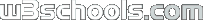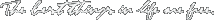Search W3Schools :
 ADVERTISEMENTS XML Certification Download XML editor Custom Programming# PHP Math Functions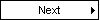## PHP Math Introduction

The math functions can handle values within the range of integer and float types.

## Installation

The math functions are part of the PHP core. There is no installation needed to use these functions.

## PHP Math Functions

PHP: indicates the earliest version of PHP that supports the function.

Function Description PHP
abs() Returns the absolute value of a number 3
acos() Returns the arccosine of a number 3
acosh() Returns the inverse hyperbolic cosine of a number 4
asin() Returns the arcsine of a number 3
asinh() Returns the inverse hyperbolic sine of a number 4
atan() Returns the arctangent of a number as a numeric value between -PI/2 and PI/2 radians 3
atan2() Returns the angle theta of an (x,y) point as a numeric value between -PI and PI radians 3
atanh() Returns the inverse hyperbolic tangent of a number 4
base_convert() Converts a number from one base to another 3
bindec() Converts a binary number to a decimal number 3
ceil() Returns the value of a number rounded upwards to the nearest integer 3
cos() Returns the cosine of a number 3
cosh() Returns the hyperbolic cosine of a number 4
decbin() Converts a decimal number to a binary number 3
dechex() Converts a decimal number to a hexadecimal number 3
decoct() Converts a decimal number to an octal number 3
deg2rad() Converts a degree to a radian number 3
exp() Returns the value of Ex 3
expm1() Returns the value of Ex - 1 4
floor() Returns the value of a number rounded downwards to the nearest integer 3
fmod() Returns the remainder (modulo) of the division of the arguments 4

getrandmax()

Returns the maximum random number that can be returned by a call to the rand() function 3
hexdec() Converts a hexadecimal number to a decimal number 3
hypot() Returns the length of the hypotenuse of a right-angle triangle 4
is_finite() Returns true if a value is a finite number 4
is_infinite() Returns true if a value is an infinite number 4
is_nan() Returns true if a value is not a number 4
lcg_value() Returns a pseudo random number in the range of (0,1) 4
log() Returns the natural logarithm (base E) of a number 3
log10() Returns the base-10 logarithm of a number 3
log1p() Returns log(1+number) 4
max() Returns the number with the highest value of two specified numbers 3
min() Returns the number with the lowest value of two specified numbers 3
mt_getrandmax() Returns the largest possible value that can be returned by mt_rand() 3
mt_rand() Returns a random integer using Mersenne Twister algorithm 3
mt_srand() Seeds the Mersenne Twister random number generator 3
octdec() Converts an octal number to a decimal number 3
pi() Returns the value of PI 3
pow() Returns the value of x to the power of y 3
rad2deg() Converts a radian number to a degree 3
rand() Returns a random integer 3
round() Rounds a number to the nearest integer 3
sin() Returns the sine of a number 3
sinh() Returns the hyperbolic sine of a number 4
sqrt() Returns the square root of a number 3
srand() Seeds the random number generator 3
tan() Returns the tangent of an angle 3
tanh() Returns the hyperbolic tangent of an angle 4

## PHP Math Constants

PHP: indicates the earliest version of PHP that supports the constant.

Constant Description PHP
M_E Returns e (approx. 2.718) 4
M_EULER Returns Euler's constant (approx. 0.577) 4
M_LNPI Returns the natural logarithm of PI (approx. 1.144) 4
M_LN2 Returns the natural logarithm of 2 (approx. 0.693) 4
M_LN10 Returns the natural logarithm of 10 (approx. 2.302) 4
M_LOG2E Returns the base-2 logarithm of E (approx. 1.442) 4
M_LOG10E Returns the base-10 logarithm of E (approx. 0.434) 4
M_PI Returns PI (approx. 3.14159) 3
M_PI_2 Returns PI/2 (approx. 1.570) 4
M_PI_4 Returns PI/4 (approx. 0.785) 4
M_1_PI Returns 1/PI (approx. 0.318) 4
M_2_PI Returns 2/PI (approx. 0.636) 4
M_SQRTPI Returns the square root of PI (approx. 1.772) 4
M_2_SQRTPI Returns 2/square root of PI (approx. 1.128) 4
M_SQRT1_2 Returns the square root of 1/2 (approx. 0.707) 4
M_SQRT2 Returns the square root of 2 (approx. 1.414) 4
M_SQRT3 Returns the square root of 3 (approx. 1.732) 4## Make your web applications look like a million bucksMost web applications today use boring methods to present data to their viewers using grids or simple HTML tables. FusionCharts induces "life" into the web applications by converting monotonous data into lively charts, gauges & maps. FusionCharts works with all technologies like ASP, ASP.NET, PHP, ColdFusion, Ruby on Rails, JSP, HTML pages etc. and connects to any database to render animated & interactive charts. It takes less than 15 minutes and no expertise whatsoever to build your first chart and just a glance of it to captivate your audience. This fact is endorsed by our 12,000 customers and 150,000 users which include a majority of the Fortune 500 companies. And yeah, your applications could look like a million bucks by spending just \$69. So go ahead, download your copy of FusionCharts and start "wow-ing" your customers now!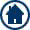HOME or Top of Page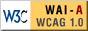Printer FriendlyW3Schools is for training only. We do not warrant the correctness of its content. The risk from using it lies entirely with the user. While using this site, you agree to have read and accepted our terms of use and privacy policy. Copyright 1999-2009 by Refsnes Data. All Rights Reserved.